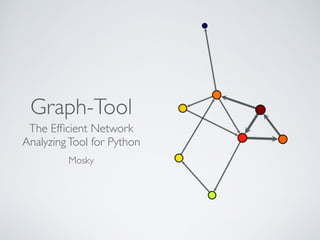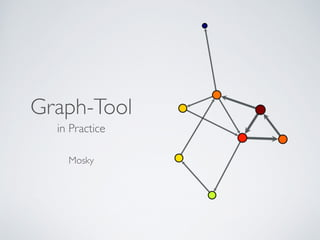Ce diaporama a bien été signalé.
Le téléchargement de votre SlideShare est en cours. ×

# Graph-Tool in Practice

Publicité
Publicité
Publicité
Publicité
Publicité
Publicité
Publicité
Publicité
Publicité
Publicité
PublicitéChargement dans…3
×

## Consultez-les par la suite

1 sur 102 Publicité

# Graph-Tool in Practice

It was the talk, titled "Graph-Tool: The Efficient Network Analyzing Tool for Python", at PyCon APAC 2014  and PyCon SG 2014 . It introduces you to Graph-Tool by mass code snippets.

 https://tw.pycon.org/2014apac
 https://pycon.sg/

It was the talk, titled "Graph-Tool: The Efficient Network Analyzing Tool for Python", at PyCon APAC 2014  and PyCon SG 2014 . It introduces you to Graph-Tool by mass code snippets.

 https://tw.pycon.org/2014apac
 https://pycon.sg/

Publicité
Publicité

### Graph-Tool in Practice

1. 1. Graph-Tool The Efﬁcient Network AnalyzingTool for Python Mosky
2. 2. Graph-Tool in Practice  Mosky
3. 3. MOSKY 3
4. 4. MOSKY • Python Charmer at Pinkoi 3
5. 5. MOSKY • Python Charmer at Pinkoi • An author of the Python packages: • MoSQL, Clime, Uniout, ZIPCodeTW, … 3
6. 6. MOSKY • Python Charmer at Pinkoi • An author of the Python packages: • MoSQL, Clime, Uniout, ZIPCodeTW, … • A speaker of the conferences • 2014: PyCon APAC, OSDC; 2013: PyCon APAC, PyConTW, COSCUP, … 3
7. 7. MOSKY • Python Charmer at Pinkoi • An author of the Python packages: • MoSQL, Clime, Uniout, ZIPCodeTW, … • A speaker of the conferences • 2014: PyCon APAC, OSDC; 2013: PyCon APAC, PyConTW, COSCUP, … • A Python instructor 3
8. 8. MOSKY • Python Charmer at Pinkoi • An author of the Python packages: • MoSQL, Clime, Uniout, ZIPCodeTW, … • A speaker of the conferences • 2014: PyCon APAC, OSDC; 2013: PyCon APAC, PyConTW, COSCUP, … • A Python instructor • mosky.tw 3
9. 9. OUTLINE 4
10. 10. OUTLINE • Introduction 4
11. 11. OUTLINE • Introduction • Create Graph 4
12. 12. OUTLINE • Introduction • Create Graph • Visualize Graph 4
13. 13. OUTLINE • Introduction • Create Graph • Visualize Graph • Analyze Graph 4
14. 14. OUTLINE • Introduction • Create Graph • Visualize Graph • Analyze Graph • Conclusion 4
15. 15. INTRODUCTION
16. 16. GRAPH-TOOL 6
17. 17. GRAPH-TOOL • It's for analyzing graph. 6
18. 18. GRAPH-TOOL • It's for analyzing graph. • Fast. It bases on   Boost Graph in C++. 6
19. 19. GRAPH-TOOL • It's for analyzing graph. • Fast. It bases on   Boost Graph in C++. • Powerful visualization 6
20. 20. GRAPH-TOOL • It's for analyzing graph. • Fast. It bases on   Boost Graph in C++. • Powerful visualization • Lot of useful algorithms 6
21. 21. GET GRAPH-TOOL 7
22. 22. GET GRAPH-TOOL • Super easy on Debian / Ubuntu • http://graph-tool.skewed.de/download#debian 7
23. 23. GET GRAPH-TOOL • Super easy on Debian / Ubuntu • http://graph-tool.skewed.de/download#debian • Super hard on Mac • http://graph-tool.skewed.de/download#macos • Install the dependencies by homebrew and pip.   Then compile it from source. • Note it may take you 3~4 hours. I warned you! 7
24. 24. CREATE GRAPH
25. 25. BEFORE STARTING 9
26. 26. BEFORE STARTING • Deﬁne your problem. 9
27. 27. BEFORE STARTING • Deﬁne your problem. • Convert it into a graphic form. 9
28. 28. BEFORE STARTING • Deﬁne your problem. • Convert it into a graphic form. • Parse raw data. 9
29. 29. MY PROBLEM 10
30. 30. MY PROBLEM • To improve the duration of an online marketplace. 10
31. 31. MY PROBLEM • To improve the duration of an online marketplace. • What's product browsing ﬂow that users prefer? 10
32. 32. IN GRAPHIC FORM 11 What Weight Vertex Product Count Edge Directed Browsing Count
33. 33. PARSING 12
34. 34. PARSING • Regular expression • Filter garbages. 12
35. 35. PARSING • Regular expression • Filter garbages. • Sorting 12
36. 36. PARSING • Regular expression • Filter garbages. • Sorting • Pickle • HIGHEST_PROTOCOL • Use tuple to save space/time. • Save into serial ﬁles. 12
37. 37. VERTEX AND EDGE import graph_tool.all as gt ! g = gt.Graph() v1 = g.add_vertex() v2 = g.add_vertex() e = g.add_edge(v1, v2) 13
38. 38. PROPERTY v_count_p = g.new_vertex_property('int') ! # store it in our graph, optionally g.vp['count'] = v_count_p 14
39. 39. FASTER IMPORT from graph_tool import Graph 15
40. 40. COUNTING name_v_map = {} for name in names: v = name_v_map.get(name) if v is None: v = g.add_vertex() v_count_p[v] = 0 name_v_map[name] = v v_count_p[v] += 1 16
41. 41. VISUALIZE GRAPH
42. 42. THE SIMPLEST gt.graph_draw( g, output_path = 'output.pdf', ) ! gt.graph_draw( g, output_path = 'output.png', ) 18
43. 43. 19
44. 44. USE CONSTANTS SIZE = 400 V_SIZE = SIZE / 20. E_PWIDTH = V_SIZE / 4. gt.graph_draw( … output_size = (SIZE, SIZE), vertex_size = V_SIZE, edge_pen_width = E_PWDITH, ) 20
45. 45. 21
46. 46. USE PROP_TO_SIZE v_size_p = gt.prop_to_size( v_count_p, MI_V_SIZE, MA_V_SIZE, ) … gt.graph_draw( … vertex_size = v_size_p, edge_pen_width = e_pwidth_p, ) 22
47. 47. 23
48. 48. USE FILL_COLOR gt.graph_draw( … vertex_fill_color = v_size_p, ) 24
49. 49. 25
50. 50. ANALYZE GRAPH
51. 51. CHOOSE AN ALGORITHM 27
52. 52. CHOOSE AN ALGORITHM • Search algorithms • BFS search … 27
53. 53. CHOOSE AN ALGORITHM • Search algorithms • BFS search … • Assessing graph topology • shortest path … 27
54. 54. CHOOSE AN ALGORITHM • Search algorithms • BFS search … • Assessing graph topology • shortest path … • Centrality measures • pagerank, betweenness, closeness … 27
55. 55. 28
56. 56. • Maximum ﬂow algorithms 28
57. 57. • Maximum ﬂow algorithms • Community structures 28
58. 58. • Maximum ﬂow algorithms • Community structures • Clustering coefﬁcients 28
59. 59. CENTRALITY MEASURES 29
60. 60. CENTRALITY MEASURES • Degree centrality • the number of links incident upon a node • the immediate risk of taking a node out 29
61. 61. CENTRALITY MEASURES • Degree centrality • the number of links incident upon a node • the immediate risk of taking a node out • Closeness centrality • sum of a node's distances to all other nodes • the cost to spread information to all other nodes 29
62. 62. 30
63. 63. • Betweenness centrality • the number of times a node acts as a bridge • the control of a node on the communication between other nodes 30
64. 64. • Betweenness centrality • the number of times a node acts as a bridge • the control of a node on the communication between other nodes • Eigenvector centrality • the inﬂuence of a node in a network • Google's PageRank is a variant of the Eigenvector centrality measure 30
65. 65. MY CHOICE 31
66. 66. MY CHOICE • Centrality measures - Closeness centrality 31
67. 67. MY CHOICE • Centrality measures - Closeness centrality • Get the products are easier to all other products. 31
68. 68. CALCULATE CLOSENESS ! ! e_icount_p = g.new_edge_property('int') e_icount_p.a = e_count_p.a.max()-e_count_p.a ! v_cl_p = closeness(g, weight=e_icount_p) ! import numpy as np v_cl_p.a = np.nan_to_num(v_cl_p.a) 32
69. 69. DRAW CLOSENESS v_cl_size_p = gt.prop_to_size( v_cl_p, MI_V_SIZE, MA_V_SIZE, ) … gt.graph_draw( … vertex_fill_color = v_cl_size_p, ) 33
70. 70. 34
71. 71. ONTHE FLY FILTERING ! v_pck_p = g.new_vertex_property('bool') v_pck_p.a = v_count_p.a > v_count_p.a.mean() ! g.set_vertex_filter(v_pck_p) # g.set_vertex_filter(None) # unset 35
72. 72. 36
73. 73. TOP N t10_idxs = v_count_p.a.argsort()[-10:][::-1] ! t1_idx = t10_idxs t1_v = g.vertex(t1_idx) t1_name = v_name_p[t1_v] t1_count = v_count_p[t1_v] 37
74. 74. SFDF LAYOUT gt.graph_draw( … pos = gt.sfdp_layout(g), ) 38
75. 75. 39
76. 76. gt.graph_draw( … pos = gt.sfdp_layout( g, eweight=e_count_p ), ) ! gt.graph_draw( … pos = gt.sfdp_layout( g, eweight=e_count_p, vweight=v_count_p ), ) 40
77. 77. 41
78. 78. 42
79. 79. 43
80. 80. FR LAYOUT gt.graph_draw( … pos = gt.fruchterman_reingold_layout(g), ) ! gt.graph_draw( … pos = gt.fruchterman_reingold_layout( g, weight=e_count_p ), ) 44
81. 81. 45
82. 82. 46
83. 83. 47
84. 84. ARF LAYOUT gt.graph_draw( … pos = gt.arf_layout(g), ) ! gt.graph_draw( … pos = gt.arf_layout( g, weight=e_count_p ), ) 48
85. 85. 49
86. 86. 50
87. 87. 51
88. 88. MY GRAPH
89. 89. 53
90. 90. CONCLUSION
91. 91. 55 CONCLUSION
92. 92. • Deﬁne problem in graphic form. 55 CONCLUSION
93. 93. • Deﬁne problem in graphic form. • Parse raw data. • Watch out!   Your data will bite you. → 55 CONCLUSION
94. 94. • Deﬁne problem in graphic form. • Parse raw data. • Watch out!   Your data will bite you. → • Visualize to understand. 55 CONCLUSION
95. 95. • Deﬁne problem in graphic form. • Parse raw data. • Watch out!   Your data will bite you. → • Visualize to understand. • Choose a proper algorithms. 55 CONCLUSION
96. 96. • Deﬁne problem in graphic form. • Parse raw data. • Watch out!   Your data will bite you. → • Visualize to understand. • Choose a proper algorithms. • Filter data which interest you. 55 CONCLUSION
97. 97. • Deﬁne problem in graphic form. • Parse raw data. • Watch out!   Your data will bite you. → • Visualize to understand. • Choose a proper algorithms. • Filter data which interest you. • Visualize again to convince. 55 CONCLUSION
98. 98. • Deﬁne problem in graphic form. • Parse raw data. • Watch out!   Your data will bite you. → • Visualize to understand. • Choose a proper algorithms. • Filter data which interest you. • Visualize again to convince. • mosky.tw 55 CONCLUSION
99. 99. DEMO
100. 100. COSCUP 2014 2014.07.19 - 2014.07.20 | Academia Sinica,Taipei,Taiwan
101. 101. LINKS • Quick start using graph-tool  http://graph-tool.skewed.de/static/doc/quickstart.html • Learn more about Graph object  http://graph-tool.skewed.de/static/doc/graph_tool.html • The possible property value types  http://graph-tool.skewed.de/static/doc/ graph_tool.html#graph_tool.PropertyMap 58
102. 102. • Graph drawing and layout  http://graph-tool.skewed.de/static/doc/draw.html • Available subpackages - Graph-Tool  http://graph-tool.skewed.de/static/doc/ graph_tool.html#available-subpackages • Centrality - Wiki  http://en.wikipedia.org/wiki/Centrality • NumPy Reference  http://docs.scipy.org/doc/numpy/reference/ 59